## How to Calculate and Solve for Linear Expansivity, Area Expansivity and Volume or Cubic Expansivity

Linear expansivity, area expansivity and volume or cubic expansivity are all parameters that are being governed by heat energy. All three parameter is dependent on temperature rise which is primarily in Celsius unit of temperature.

Linear expansivity additionally depends on the original length and final length, area expansivity additionally depends on the original area and final area and lastly, cubic or volume expansivity depends on the original volume and final volume.

The formula for calculating linear expansivity is (l2 – l1) / l1θ
l2 represents the final length.
l1 represents the original length.
θ represents the temperature rise in Celsius.

The formula for calculating area expansivity is (A2 – A1) / A1θ
A2 represents the final area.
A1 represents the original area.
θ represents the temperature rise in Celsius.

The formula for calculating volume or cubic expansivity is (V2 – V1) / V1θ
V2 represents the final volume.
V1 represents the original volume.
θ represents the temperature rise in Celsius.

## Nickzom Calculator Solves Molar Concentration in Chemistry

According to Wikipedia,

Molar concentration (also called molarityamount concentration or substance concentration) is a measure of the concentration of a chemical species, in particular of a solute in a solution, in terms of amount of substance per unit volume of solution. In chemistry, the most commonly used unit for molarity is the number of moles per litre, having the unit symbol mol/L. A solution with a concentration of 1 mol/L is said to be 1 molar, commonly designated as 1 M.

Nickzom Calculator+ (Professional Version) – The Calculator Encyclopedia requires two parameters to get the answer for a molar concentration in Chemistry such as:

• Number of Moles
• Volume

One can access Nickzom Calculator+ (Professional Version) – The Calculator Encyclopedia via any of these channels:

First, go to the calculator map, scroll down to the Chemistry section and click on Basic Chemistry.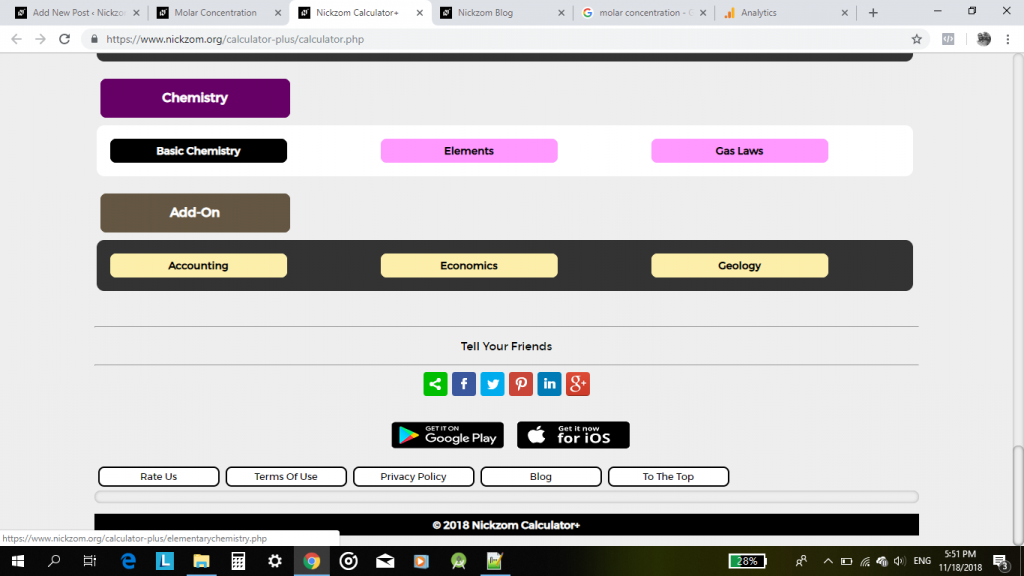Now, click on Molar Concentration in Chemistry

## Nickzom Calculator Solves Calculations on Gas Laws

In Physics and Chemistry, there are four prominent gas laws that govern calculations related to gases. These laws have major parameters such as:

• Pressure
• Temperature
• Volume

The four gas laws are:

• Boyle’s Law
• Charles’ Law
• Pressure or Gay Lussac’s Law
• The General Gas Equation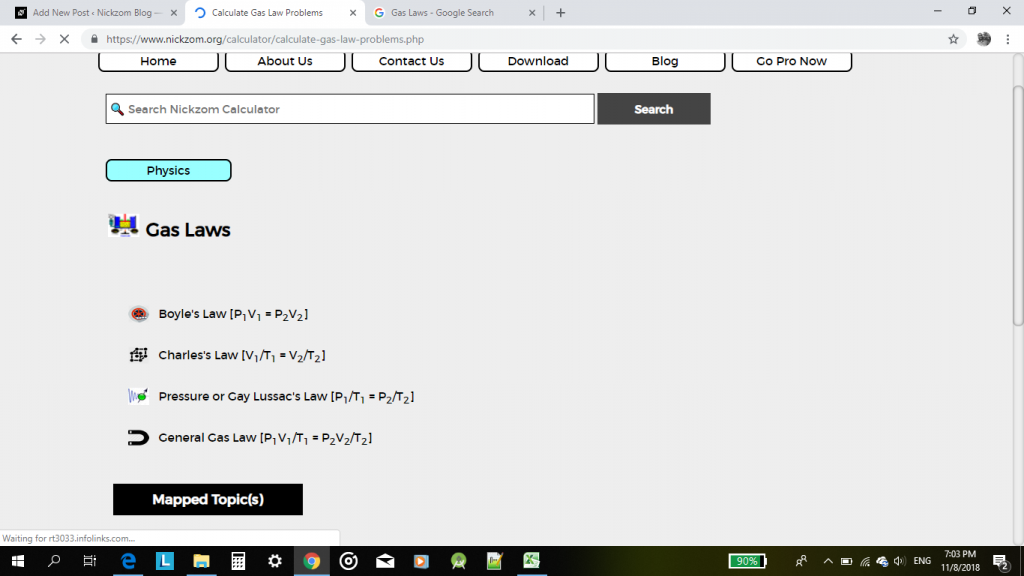According to Boyle’s law, pressure is indirectly proportional to volume.

According to Charles’ law, volume is directly proportional to temperature.

According to Pressure or Gay Lussac’s law, pressure is directly proportional to temperature.

According to the General Gas Equation or law, the product of pressure and volume is directly proportional to temperature.

For this post, I would be given an example of how Nickzom Calculator can solve a problem on gas laws with the general gas equation or law.

One can access Nickzom Calculator via any of these channels:

Let’s assume that one is looking for an unknown temperature.

First and foremost, click on General Gas Law.

Then click on Unknwon Temperature.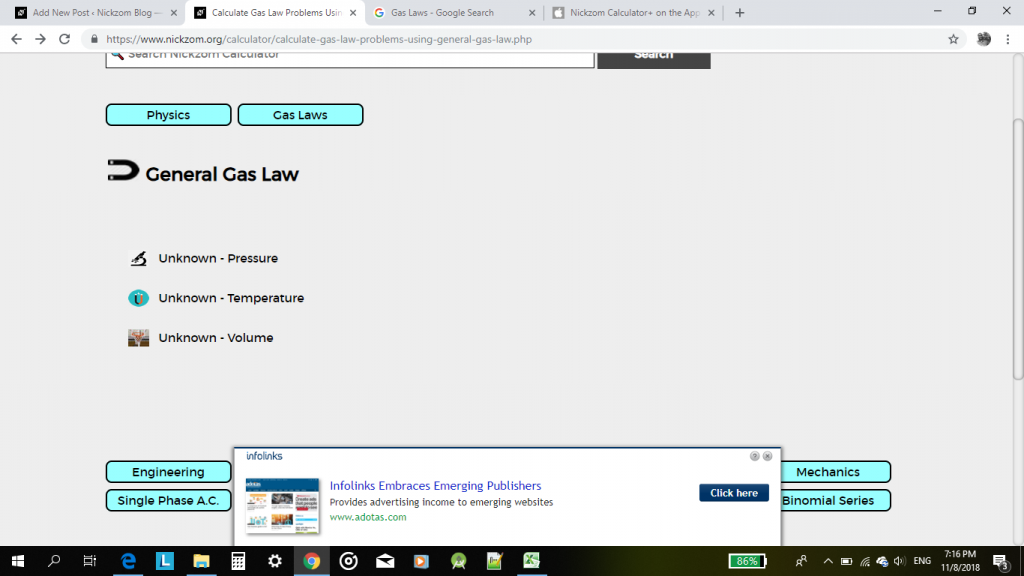Now, proceed to enter the values of the known parameters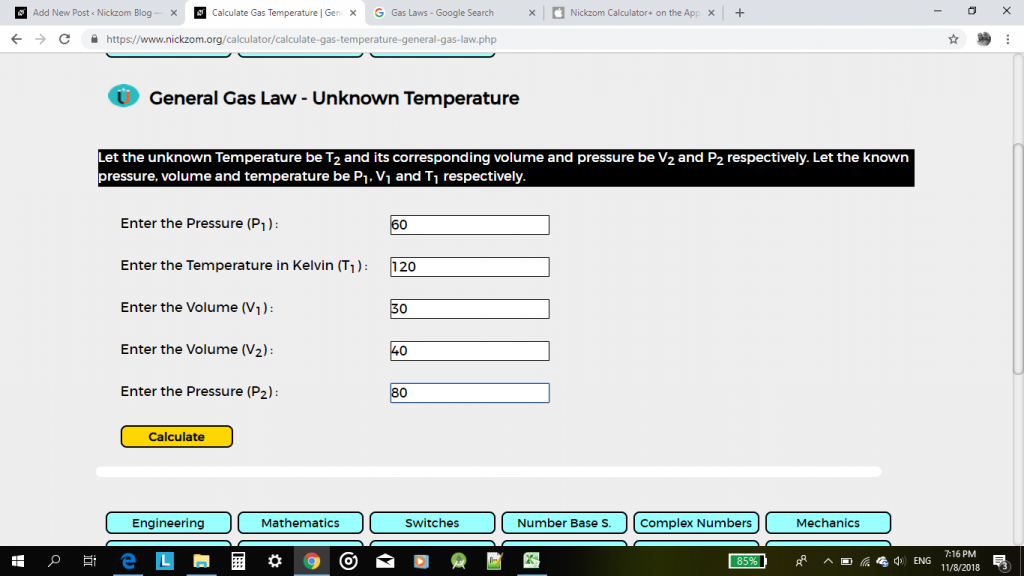Then, click on calculate.

The result would be displayed for you in less than a second.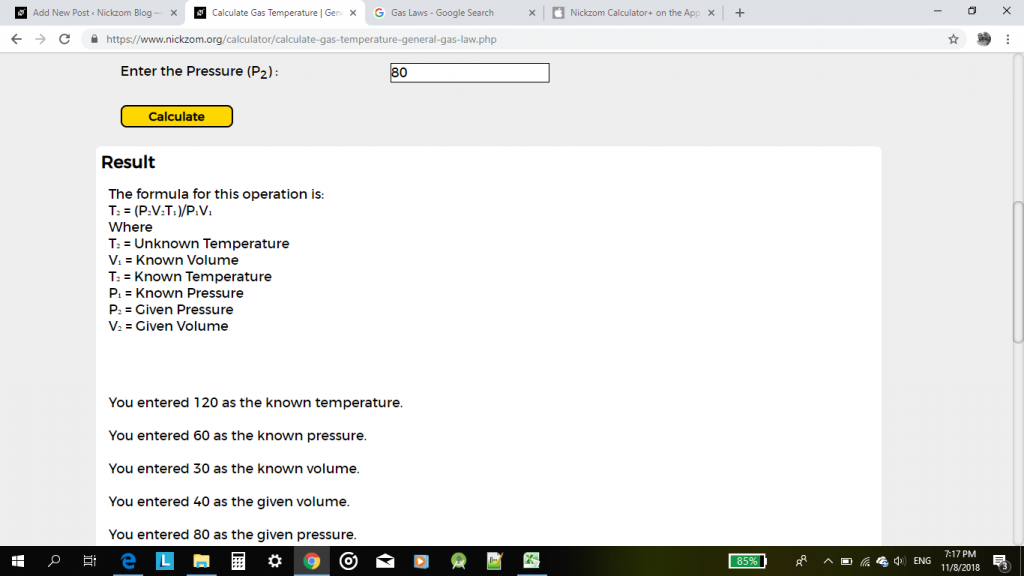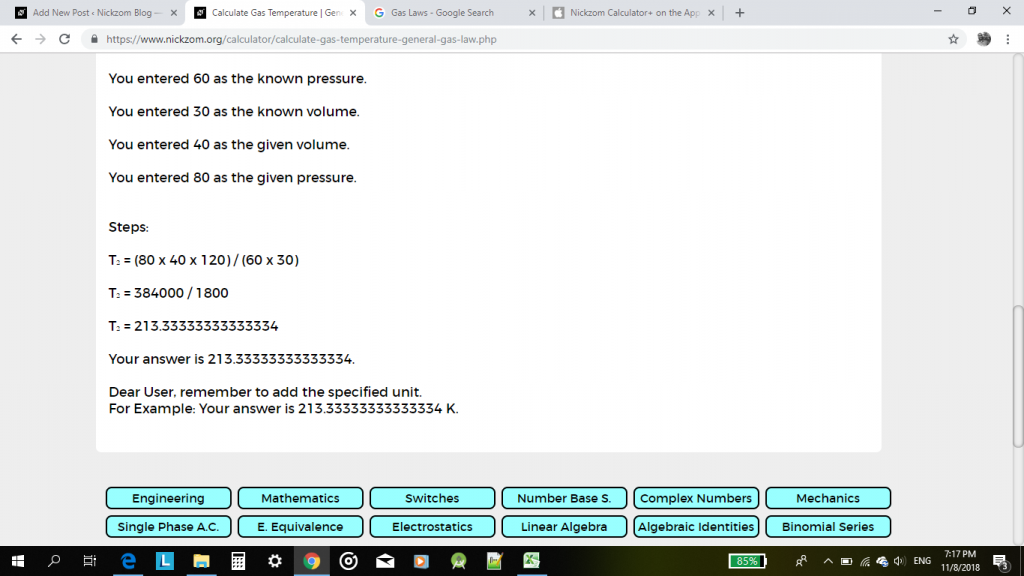This pattern can be used to solve any calculation problem one faces in gas laws.